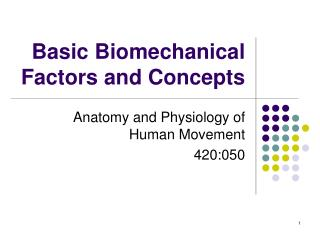# Basic Biomechanical Factors and Concepts - PowerPoint PPT PresentationDownload PresentationBasic Biomechanical Factors and Concepts

Basic Biomechanical Factors and ConceptsDownload Presentation## Basic Biomechanical Factors and Concepts

- - - - - - - - - - - - - - - - - - - - - - - - - - - E N D - - - - - - - - - - - - - - - - - - - - - - - - - - -
##### Presentation Transcript

1. Basic Biomechanical Factors and Concepts Anatomy and Physiology of Human Movement 420:050

2. Outline • Levers • Anatomical Levers

3. Outline • Levers • Anatomical Levers

4. Introduction to Levers • Lever: Simple machine that operates according to principle of torques • Torque: The turning effect of a force T = Fd

5. d How can you change torque? 1. Change F 2. Change d 3. Change direction of F F

6. d Optimal direction = 90 degrees F

7. Function of Levers • Two functions: 1. Force 2. Speed/ROM

8. Function of Levers • Force • Examples? • Common traits? • Rigid bar • Fixed point • Lever movement vs. resistance movement

9. R F

10. Function of Levers • Speed/ROM • Examples? • Common traits? • Rigid bar • Fixed point • Lever movement vs. resistance movement

11. R F

12. Components of a Lever System • Lever: Rigid bar • Fulcrum: Axis of rotation/fixed point • Force: • Applied force (F) • Resistance force (R) • Moment arm: d • Applied force • Resistance

13. More Concepts • Mechanical advantage • Levers designed for force • Mechanical disadvantage • Levers designed for speed/ROM

15. Advantage: Small effort moves big resistance Disadvantage: Big movement required to move resistance a small distance

16. Human Application? • Lever? Bones (Not always a bar) • Fulcrum? Joints • Applied force? Muscles • Resistance force? Weight of limb, external resistance

17. Classification of Levers • Lever classification based on the relative location of: 1. Axis of rotation/fulcrum (A) 2. Resistance force (R) 3. Applied force (F) A R F

18. First Class Lever • Center: (A) – Axis of rotation/fulcrum • Mechanical advantage • Mechanical disadvantage • Examples Crow bar Seesaw

20. Second Class Lever • Center: (R) – Resistance force • Mechanical advantage • Always • Mechanical disadvantage • Never • Examples Wheelbarrow Nutcracker

21. Third Class Lever • Center: (F) – Applied force • Mechanical advantage • Never • Mechanical disadvantage • Always • Examples Rowing Shoveling Bat, tennis racket

22. Baseball, tennis?

23. Human Application • First class lever • Elbow extension against a resistance

24. Mechanical advantage or disadvantage (R) – Resistance force (F) – Applied force (A) – Axis of rotation/fulcrum

25. Human Application • Second class lever • Ankle plantar flexion